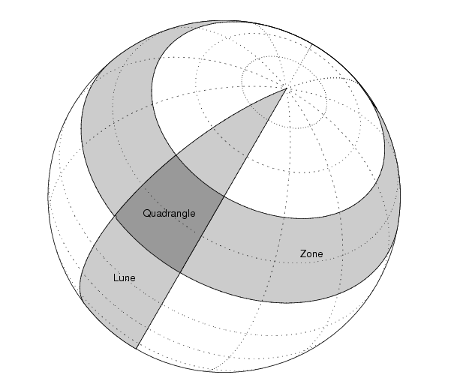## Syntax

``a = areaquad(lat1,lon1,lat2,lon2)``
``a = areaquad(lat1,lon1,lat2,lon2,ellipsoid)``
``a = areaquad(___,units)``

## Description

example

````a = areaquad(lat1,lon1,lat2,lon2)` calculates the surface area of the latitude-longitude quadrangle bound by the parallels `lat1` and `lat2` and the meridians `lon1` and `lon2`. This syntax references the coordinates to a unit sphere and returns `a` as the fraction of the sphere that the quadrangle covers.```

example

````a = areaquad(lat1,lon1,lat2,lon2,ellipsoid)` specifies the reference ellipsoid for the parallels and meridians. This syntax returns the area in square units using the units of the semimajor axis of the reference ellipsoid.```
````a = areaquad(___,units)` specifies the angle units for the parallels and meridians.```

## Examples

collapse all

Find the surface area of the quadrangle bound by the parallels 30ºN and 45ºN and the meridians 25ºW and 60ºE. When you do not specify a reference ellipsoid as input, the `areaquad` function references the quadrangle to the unit sphere and returns the area as the fraction of the sphere that the quadrangle covers, where `1` is the entire sphere.

`a = areaquad(30,-25,45,60)`
```a = 0.0245 ```

This quadrangle covers approximately 2.45% of the unit sphere.

Create a World Geodetic System of 1984 (WGS84) reference ellipsoid with a length unit of kilometers.

`wgs84 = wgs84Ellipsoid("km");`

Find the area of the quadrangle that is bound by the parallels 30ºN and 45ºN and the meridians 25ºW and 60ºE. When you specify a reference ellipsoid as input, the `areaquad` function returns area in square units using the length unit of the ellipsoid.

`a = areaquad(30,-25,45,60,wgs84)`
```a = 1.2477e+07 ```

## Input Arguments

collapse all

Latitude of the first parallel, specified as a scalar, vector, matrix, or N-D array.

When you specify a vector, matrix, or N-D array, each element specifies the latitude of the first parallel of a separate quadrangle.

The sizes of `lat1`, `lon1`, `lat2`, and `lon2` must match.

Data Types: `double`

Longitude of the first meridian, specified as a scalar, vector, matrix, or N-D array.

When you specify a vector, matrix, or N-D array, each element specifies the longitude of the first meridian of a separate quadrangle.

The sizes of `lat1`, `lon1`, `lat2`, and `lon2` must match.

Data Types: `double`

Latitude of the second parallel, specified as a scalar, vector, matrix, or N-D array.

When you specify a vector, matrix, or N-D array, each element specifies the latitude of the second parallel of a separate quadrangle.

The sizes of `lat1`, `lon1`, `lat2`, and `lon2` must match.

Data Types: `double`

Longitude of the second meridian, specified as a scalar, vector, matrix, or N-D array.

When you specify a vector, matrix, or N-D array, each element specifies the longitude of the second meridian of a separate quadrangle.

The sizes of `lat1`, `lon1`, `lat2`, and `lon2` must match.

Data Types: `double`

Reference ellipsoid, specified as a `referenceSphere` object, a `referenceEllipsoid` object, an `oblateSpheroid` object, or a two-element vector of the form `[semimajor_axis eccentricity]`, where `semimajor_axis` is the length of the semimajor axis and `eccentricity` is the eccentricity. The values `semimajor_axis` and `eccentricity` must be of data type `double`.

The default value of `[1 0]` represents the unit sphere.

Angle units of the parallels and meridians, specified as one of these options:

• `"degrees"` — Degrees

• `"radians"` — Radians

Data Types: `char` | `string`

## Output Arguments

collapse all

Surface area of the quadrangle, returned as a scalar, vector, matrix, or N-D array of the same size as `lat1`, `lon1`, `lat2`, and `lon2`. Each element of `a` is the area for the quadrangle defined by the corresponding elements of `lat1`, `lon1`, `lat2`, and `lon2`.

When you specify the `ellipsoid` argument, the area is in square units using the units of the semimajor axis of the reference ellipsoid. Otherwise, the area is the fraction of the unit sphere that the quadrangle covers.

collapse all

A latitude-longitude quadrangle is a region bounded by two meridians and two parallels. In spherical geometry, a quadrangle is the intersection of a lune (a section bounded by two meridians) and a zone (a section bounded by two parallels).## Algorithms

• The `areaquad` function uses spherical geometry and returns exact calculations.

• When you specify `ellipsoid` as a nonspherical ellipsoid, the function converts the latitude data to the auxiliary authalic sphere.

## Version History

Introduced before R2006a